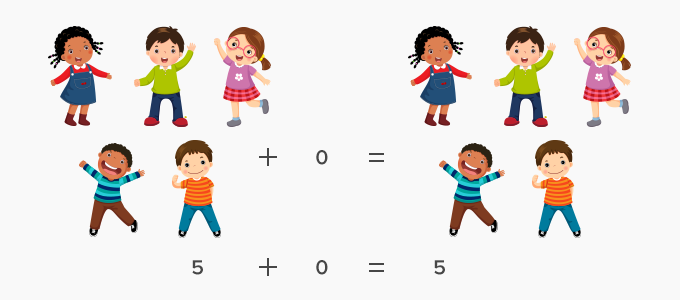# Additive Identity Property Of 0 - Definition with Examples

The Complete K-5 Math Learning Program Built for Your Child

• 30 Million Kids

Loved by kids and parent worldwide

• 50,000 Schools

Trusted by teachers across schools

• Comprehensive Curriculum

Aligned to Common Core

Additive identity is one of the properties of addition. The addition is the process of taking two or more numbers and adding them together. In other words, it is the total sum of all the numbers.

There are four mathematical properties of addition. These are:

• Closure Property

• Commutative Property

• Associative Property

Here, we will discuss the additive identity.

Additive identity is a number, which when added to any number, gives the sum as the number itself. It means that additive identity is “0” as adding 0 to any number, gives the sum as the number itself.

Example:

2 + 0 = 2

0 + 5 = 5

For any set of numbers, that is, all integers, rational numbers, complex numbers, the additive identity is 0. It is because when you add 0 to any number; it doesn’t change the number and keeps its identity.

Therefore, a + 0 = 0 + a = a

Where, a is any number.

However, additive identity cannot be associated to natural numbers, since 0 is not considered as a natural number. Natural numbers start from 1.

Let us see some examples to understand additive identity.

There are 5 kids on a team. No other kid joined the team till the end of the game. How many kids were in the game?

As no one joined the game, this means there was no change in the number of kids.

5 kids + 0 kids = 5 kidsA flock of 12 birds sat over the branch of a tree. No more birds joined them. How many birds are sitting on the branch of the tree?

As no bird joined the flock of 12 birds, hence the number of birds remained the same.

This can be written in a mathematical expression as

12 birds  + 0 birds  = 12 birdsFun Facts The additive identity property is also called the zero property of addition.

Won Numerous Awards & Honors